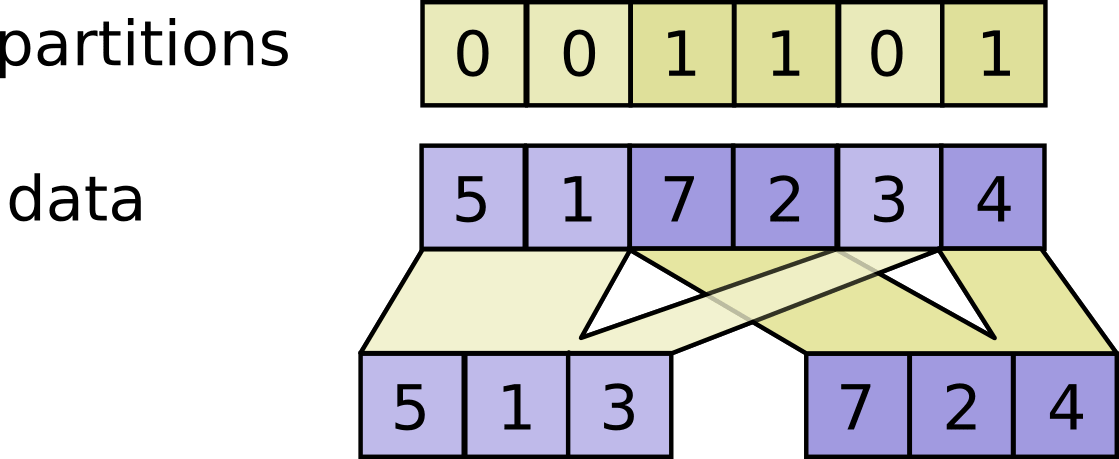/TensorFlow 2.4

# tf.raw_ops.DynamicPartition

Partitions `data` into `num_partitions` tensors using indices from `partitions`.

For each index tuple `js` of size `partitions.ndim`, the slice `data[js, ...]` becomes part of `outputs[partitions[js]]`. The slices with `partitions[js] = i` are placed in `outputs[i]` in lexicographic order of `js`, and the first dimension of `outputs[i]` is the number of entries in `partitions` equal to `i`. In detail,

```outputs[i].shape = [sum(partitions == i)] + data.shape[partitions.ndim:]

outputs[i] = pack([data[js, ...] for js if partitions[js] == i])
```

`data.shape` must start with `partitions.shape`.

#### For example:

```# Scalar partitions.
partitions = 1
num_partitions = 2
data = [10, 20]
outputs = []  # Empty with shape [0, 2]
outputs = [[10, 20]]

# Vector partitions.
partitions = [0, 0, 1, 1, 0]
num_partitions = 2
data = [10, 20, 30, 40, 50]
outputs = [10, 20, 50]
outputs = [30, 40]
```

See `dynamic_stitch` for an example on how to merge partitions back.Args
`data` A `Tensor`.
`partitions` A `Tensor` of type `int32`. Any shape. Indices in the range `[0, num_partitions)`.
`num_partitions` An `int` that is `>= 1`. The number of partitions to output.
`name` A name for the operation (optional).
Returns
A list of `num_partitions` `Tensor` objects with the same type as `data`.